# Spanning Sets

A Spanning Set is the smallest set of vectors where any vector in a certain space can be represented as a Linear Combination of the spanning set vectors.

The Spanning Set can span a line (1-dimension), a plane (2-dimension) or a space (3-dimensions).

Look how the Standard Basis unit vectors can be used to create Spanning Set:

 Spanning Set Line Spanning Set Plane Spanning Set Space $$\hat{\imath}$$ x-dir $$\hat{\imath}$$, $$\hat{\jmath}$$ xy-plane $$\hat{\imath}$$, $$\hat{\jmath}$$, $$\hat{k}$$ 3D space $$\hat{\jmath}$$ y-dir $$\hat{\imath}$$, $$\hat{k}$$ xz-plane $$\hat{k}$$ z-dir $$\hat{\jmath}$$, $$\hat{k}$$ yz-plane

Given a spanning set of just $$\hat{\imath}$$, the only vectors we can make using linear combnations are vectors along the x-axis. Therefore, it spans a line.

$$2\hat{\imath}$$,$$-1.5\hat{\imath}$$, etc.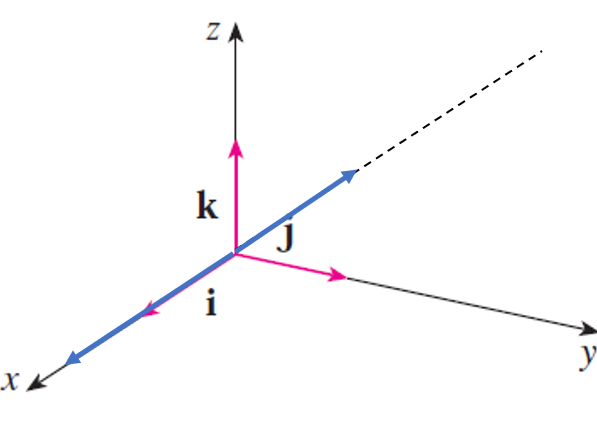Given a spanning set of $$\hat{\jmath}$$, $$\hat{k}$$, the only vectors we can make using linear combinations are vectors in the yz-plane. Therefore, they span a plane.

$$2\hat{\jmath} + \hat{k}$$, $$-2\hat{\jmath} + \hat{k}$$, etc.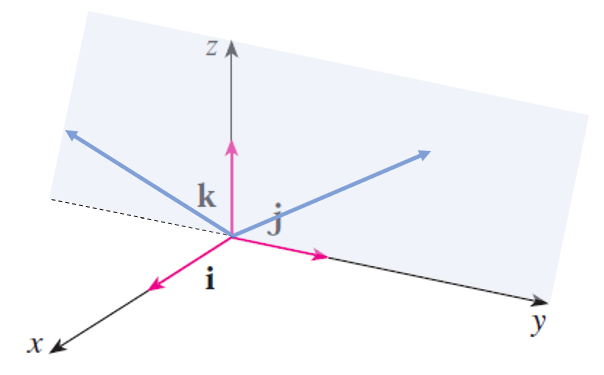Given a spanning set of $$\hat{\imath}$$, $$\hat{\jmath}$$, $$\hat{k}$$, we can make any vector in 3D space using linear combinations. Therefore, they space a space.

$$\hat{\imath} + \hat{\jmath} - 0.5\hat{k}$$, etc.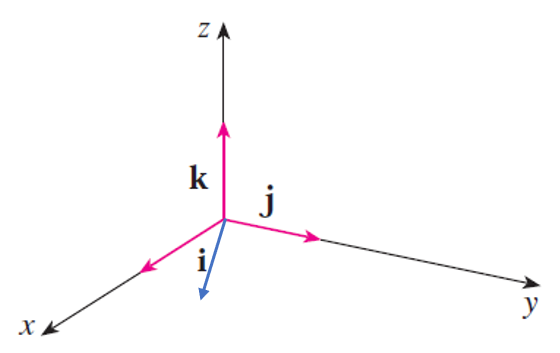What is the minimum number of vectors to span a plane?

What is the minimum number of vectors to span a plane from $$\left<10, 4 \right>$$, $$\left<1, 0 \right>$$ and $$\left<0, 1 \right>$$?

Let's see a few more examples: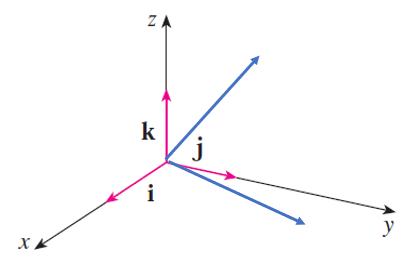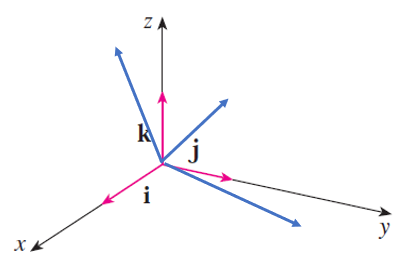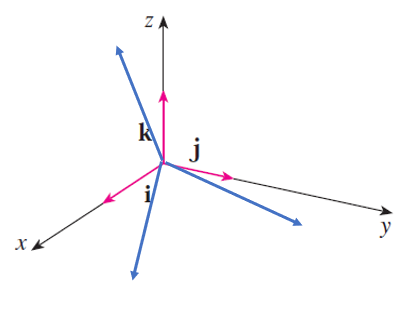The lines are Coplanar. They both lie on a plane. The lines are Coplanar. They all lie on a plane. The lines are non-Coplanar. They all cannot lie on the same plane.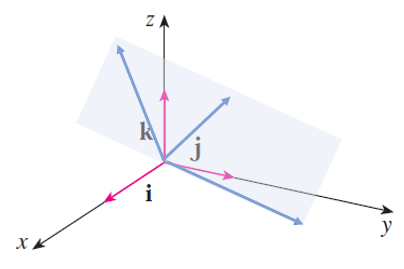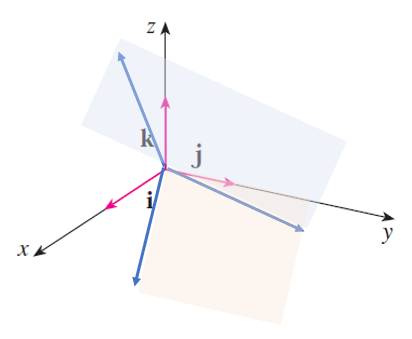Spans a plane. Span a plane. Span a space.

What do the following vectors span? $$\vec{u} = \left<3,-1,4 \right>, \vec{v} = \left<6,-4,-8\right>, \vec{w} = \left<7,-3,4 \right>$$